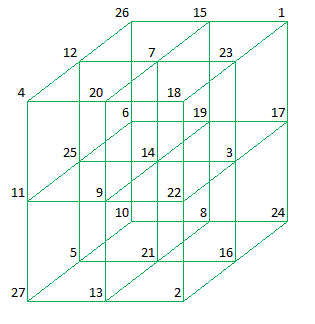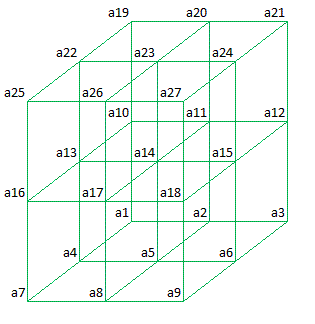Office Applications and Entertainment, Magic CubesIndex About the Author

 1.0 Introduction Comparable with Magic Squares as discussed on this Website, Magic Cubes of order n can be defined as arrangements of n3 numbers, usually distinct integers, in cubes, such that the n numbers in all rows, columns, pillars and all or a part of the plane - and space diagonals sum to the same constant. A normal Magic Cube contains the integers from 1 to n3. The constant sum in every row, column, pillar and diagonal is called the Magic Constant or Magic Sum s1. The Magic Constant of a normal Magic Cube depends only on n and has the value s1 = n(n3 + 1)/2. Section 2.1 below describes how Magic Cubes can be constructed by means of elementary analytical methods used in solving couples of linear equations. 2.0 Magic Cubes (3 x 3 x 3) 2.1 Historical Background The first 3 x 3 x 3 Magic Cube was published by T. Hugel in 1876. All rows, columns, pillars and 4 space diagonals sum to 42. The cube and the three horizontal planes of this cube are shown below:Plane 3

 26 15 1 12 7 23 4 20 18

Plane 2

 6 19 17 25 14 3 11 9 22

Plane 1

 10 8 24 5 21 16 27 13 2
 Plane 1, 2 and 3 are respectively the bottom -, the middle - and the top square. It should be noted that Plane 1 and 3 are semi magic and only Plane 2 is magic. 2.2 Analytic Solution In general Magic Cubes of order 3 can be represented as follows:Plane 3

 a19 a20 a21 a22 a23 a24 a25 a26 a27

Plane 2

 a10 a11 a12 a13 a14 a15 a16 a17 a18

Plane 1

 a1 a2 a3 a4 a5 a6 a7 a8 a9
 As the numbers a(i), i = 1 ... 27, in all rows, columns, pillars and space diagonals sum to the same constant this results in following linear equations:
 Rows Columns Diagonals Plane 1 a1 + a4 + a7 = 42 a2 + a5 + a8 = 42 a3 + a6 + a9 = 42 a1 + a2 + a3 = 42 a4 + a5 + a6 = 42 a7 + a8 + a9 = 42 Plane 2 a10 + a13 + a16 = 42 a11 + a14 + a17 = 42 a12 + a15 + a18 = 42 a10 + a11 + a12 = 42 a13 + a14 + a15 = 42 a16 + a17 + a18 = 42 a10 + a14 + a18 = 42 a12 + a14 + a16 = 42 Plane 3 a19 + a22 + a25 = 42 a20 + a23 + a26 = 42 a21 + a24 + a27 = 42 a19 + a20 + a21 = 42 a22 + a23 + a24 = 42 a25 + a26 + a27 = 42 Pillars a1 + a10 + a19 = 42 a4 + a13 + a22 = 42 a7 + a16 + a25 = 42 a2 + a11 + a20 = 42 a5 + a14 + a23 = 42 a8 + a17 + a26 = 42 a3 + a12 + a21 = 42 a6 + a15 + a24 = 42 a9 + a18 + a27 = 42 Space Diagonals a1 + a14 + a27 = 42 a7 + a14 + a21 = 42 a3 + a14 + a25 = 42 a9 + a14 + a19 = 42
 which results in following minimum number of linear equations: a(25) =  42 - a(26) - a(27) a(22) =  42 - a(23) - a(24) a(21) =  42 - a(24) - a(27) a(20) =  42 - a(23) - a(26) a(19) = -42 + a(23) + a(24) + a(26) + a(27) a(18) = -28 + a(23) + a(24) + a(26) a(17) =  56 - a(23) - 2 * a(26) a(16) =  14 - a(24) + a(26) a(15) =  56 - a(23) - 2 * a(24) a(14) =  14 a(13) = -28 + a(23) + 2 * a(24) a(12) =  14 + a(24) - a(26) a(11) = -28 + a(23) + 2 * a(26) a(10) =  56 - a(23) - a(24) - a(26) a( 9) =  70 - a(23) - a(24) - a(26) - a(27) a( 8) = -14 + a(23) + a(26) a( 7) = -14 + a(24) + a(27) a( 6) = -14 + a(23) + a(24) a( 5) =  28 - a(23) a( 4) =  28 - a(24) a( 3) = -14 + a(26) + a(27) a( 2) =  28 - a(26) a( 1) =  28 - a(27) Abovementioned reduction is achieved by means of a VBA for Excel procedure (Algorithm3), based on reduction algorithms as described in “Analytische Meetkunde II” by Dr. J. Bijl & Drs. W. J. H. Salet. It should be noted that the same set of linear equations will result, when the equations for the diagonals of Plane 2 are omitted. So the fact that Plane 2 is a Magic Square is not a defining property, but a consequence of the defining properties that all rows, columns, pillars and space diagonals sum to the Magic Constant (42). The linear equations shown above, also generated by this procedure, are ready to be solved by the routine MgcCube3 which will finally generate all possible Magic Cubes of order 3 for the defined Magic Constant. The solutions are obtained by guessing a(23), a(24), a(26) and a(27) and filling out these guesses in the abovementioned equations which results in 192 Magic Cubes of the 3th order within 2.75 seconds. The generated solutions of the 3 x 3 x 3 Magic Cubes, for integers from 1 to 27 and related Magic Constant 42, are shown in Attachment 1, showing all solutions, including those which can be obtained from one of the others by rotation and/or reflection. 2.3 Rotation and Reflection Rotation: In general a cube has 4 x 6 = 24 orientations. For Cube001 these orientations are shown in Attachment 2. Reflection: Without jeopardizing the properties of a Magic Cube, the sequence of the planes can be reversed in either of the three directions. This operation is further referred to as reflection (ref. Attachment 3). Combined Rotation and Refelction: If the sets of orientations (24) of the cubes mentioned above (8) are compared, it appears that only two sets contain different cubes. Consequently each Magic Cube is part of a Class of 2 x 24 = 48 Magic Cubes. By means of elimination, 4 (= 192/48) Base Cubes can be found to cover all 192 Magic Cubes shown in Attachment 1. A set of possible Base Cubes is shown in Attachment 4. 2.4 Spreadsheet Solution The linear equations deducted in Section 2.2 above, can be applied in an Excel spreadsheet (ref. CnstrSngl3). Only the red figures have to be “guessed” to construct a Magic Cube of the 3th order (wrong solutions are obvious).Index About the Author